Latest Banking jobs   »

# Quantitative Aptitude Quiz For SBI PO Prelims 2022- 14th December

Q1. Find the probability of 7 letters word that can be formed from the letters of the word ‘CASTING’ so that vowels always come together.
(a) 2/7
(b) 19/42
(c) 4/15
(d) 10/21
(e) 5/14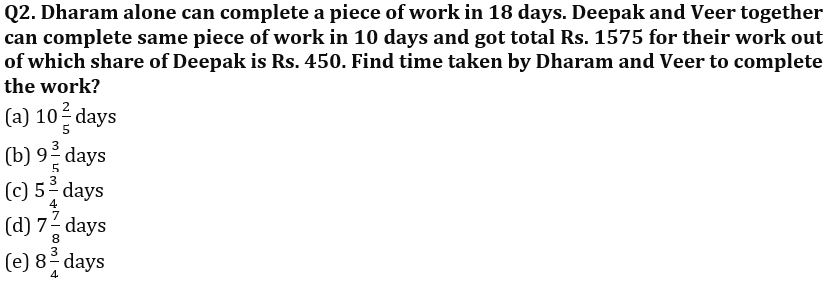Q3. Ratio of volume of conical tent to total surface area of hemispherical bowl is 8 : 1. Radius of bowl and tent is equal and slant height of tent is 75cm. If radius of conical tent is equal to side of a cube, then find curved surface area of cube.
(a) 1576 cm²
(b) 1764 cm²
(c) 1852 cm²
(d) 1690 cm²
(e) 1512 cm²

Q4. Train-A is heading towards city-Y from city-X and train-B is heading towards city-X from city-Y. Train-A left city-X at 6 : 00 A.M. and train-B left city-Y at 10 : 00 A.M. and speed of train-B is 80% more than that of train-A and both trains reach their respective destination at same time. If train-B meets train-A after running for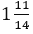hours and speed of train-A is 60km/hr., then find at what time both trains reached their respective destinations?
(a) 1 : 00 P.M.
(b) 2 : 00 P.M.
(c) 3 : 00 P.M.
(d) 6 : 00 P.M.
(e) 4 : 00 P.M.

Q5. ‘A’ purchased two beds at same price. He further sold one bed to ‘B’ at 60% profit and other bed to ‘C’ at 20% profit. ‘C’ further sold the bed to ‘D’ at 20% less than the average of cost price of bed for ‘B’ & ‘C’. If D incurred 25% loss by selling the bed to ‘E’ at Rs.2100, then find the difference between the cost price of a bed for ‘A’ and ‘D’.
(a) Rs.700
(b) Rs.450
(c) Rs.900
(d) Rs.300
(e) Rs.600

Q6. Ratio of present age of Veer, Sameer, Divyaraj, Ayush and Sumit is 14 : 15 : 13 : 12 : 16 and sum of age of Veer, Divyaraj & Sumit four years hence will be 44 years more than sum of present age of Sameer & Ayush. Find the ratio of age of Veer, Sameer, Divyaraj, Ayush and Sumit after 10 years?
(a) 19 : 20 : 18 : 16 : 21
(b) 19 : 20 : 16 : 17 : 21
(c) 19 : 20 : 22 : 17 : 21
(d) 19 : 20 : 18 : 17 : 21
(e) None of these

Q7. A vessel contains mixture in which 25% is water and rest is milk. If 40 liters more water added in vessel, then quantity of water becomes 40% of mixture. Find the quantity of milk in the vessel?
(a) 100 l
(b) 140 l
(c) 160 l
(d) 120 l
(e) 80 l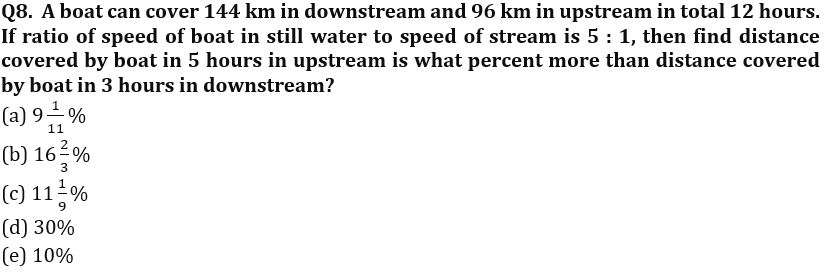Q9. Radius of smaller circular park is 60 m and area of bigger circular park is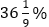more than area of smaller circular park. If side of a square park is half of the radius of bigger circular park and cost of fencing the square park is 16 per m, then find the total cost of fencing the square park?
(a) 2200 Rs.
(b) 2240 Rs.
(c) 2280 Rs.
(d) 2260 Rs.
(e) None of these

Q10. Four men and four women can complete a piece of work in 5 days, while six men & 8 women can complete the same work in 3 days. If ten men and ‘x’ women start three times of the previous work and complete it in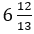day, then find ‘x’?
(a) 4
(b) 2
(c) 8
(d) 6
(e) 10

Q11.Train – A crosses train – B in 4 seconds while running in opposite direction and train – B crosses a pole in 3 seconds. Length of train – B is 60 meters. If ratio of speed of train – A to speed of train – B is 5 : 8, then find the time taken by train – B to cross train – A while running in same direction.
(a) 17⅓ seconds
(b) 15⅔ seconds
(c) 11⅓ seconds
(d) 12⅔ seconds
(e) 10⅔ seconds

Q12. A solid is in the form of a cylinder with hemispherical ends. If the height of the solid is 26cm and diameter of cylindrical part is 14cm, then find the cost of covering the solid with metal sheet (Cost of metal sheet is Rs.15/cm²).
(a) Rs.16570
(b) Rs.15440
(c) Rs.17160
(d) Rs.12420
(e) Rs.14370

Q13. Vessel-A contains 80 liters mixture of petrol and kerosene oil in the ratio 3 : 1 and vessel-B contains mixture of diesel, petrol and kerosene oil in the ratio 1 : 3 : 5. Mixtures of both vessels are mixed in another vessel-C and quantity of petrol in vessel-C is 10 liters more than the quantity of kerosene oil in vessel-C. Then, find the capacity of vessel-B.
(a) 140 liters
(b) 135 liters
(c) 120 liters
(d) 125 liters
(e) 130 liters

Q14. Bag-A contains 6 blue balls, 7 red balls and 2 green balls and Bag-B contains 5 blue balls, x red balls and 2 green balls. A bag is chosen randomly and two balls are taken out from it randomly, then the probability of getting two red balls is 2/15. Find number of red balls in Bag-B.
(a) 2
(b) 5
(c) 1
(d) 4
(e) None of the above.

Q15. There are three pipes-A, B & C. A & B are filling pipes and C is emptying pipe. Pipe-A alone can fill the tank in 1 hour & pipe-C is 20% more efficient than pipe-A. If pipe-A & B working together fill the tank in 75/2 minutes, then find in how much time pipe-A, B & C working together can fill the tank?
(a) 6 hours
(b) 4.5 hours
(c) 2.5 hours
(d) 3 hours
(e) 5 hours

Solutions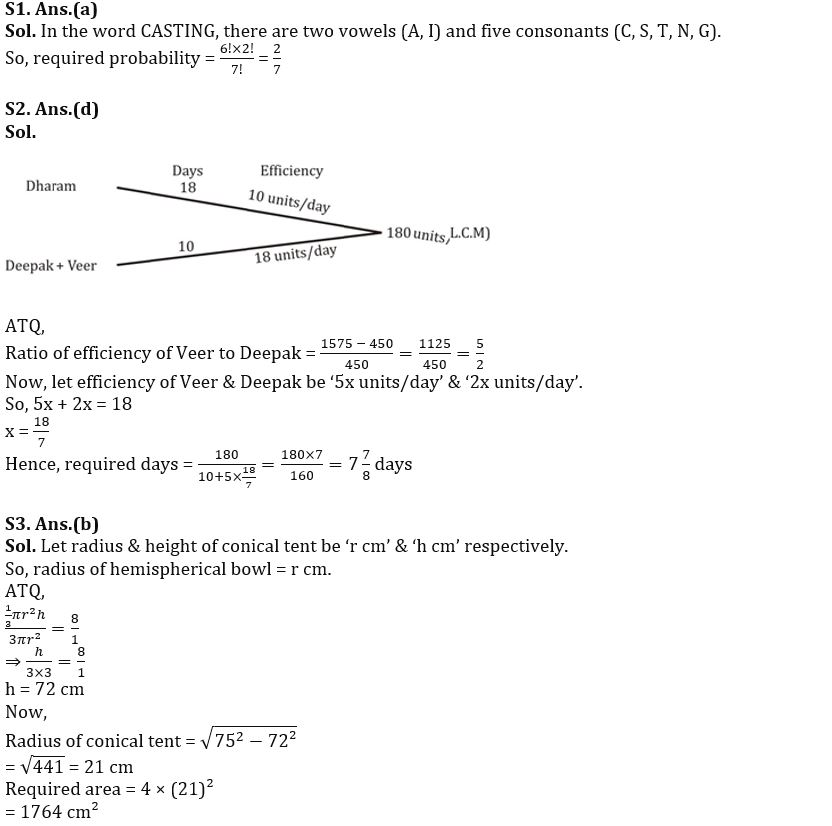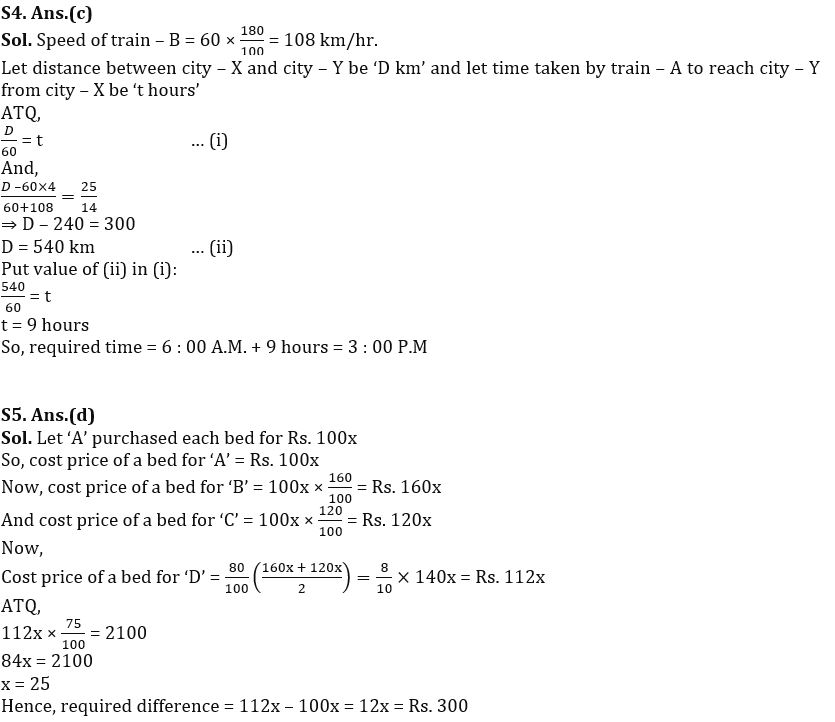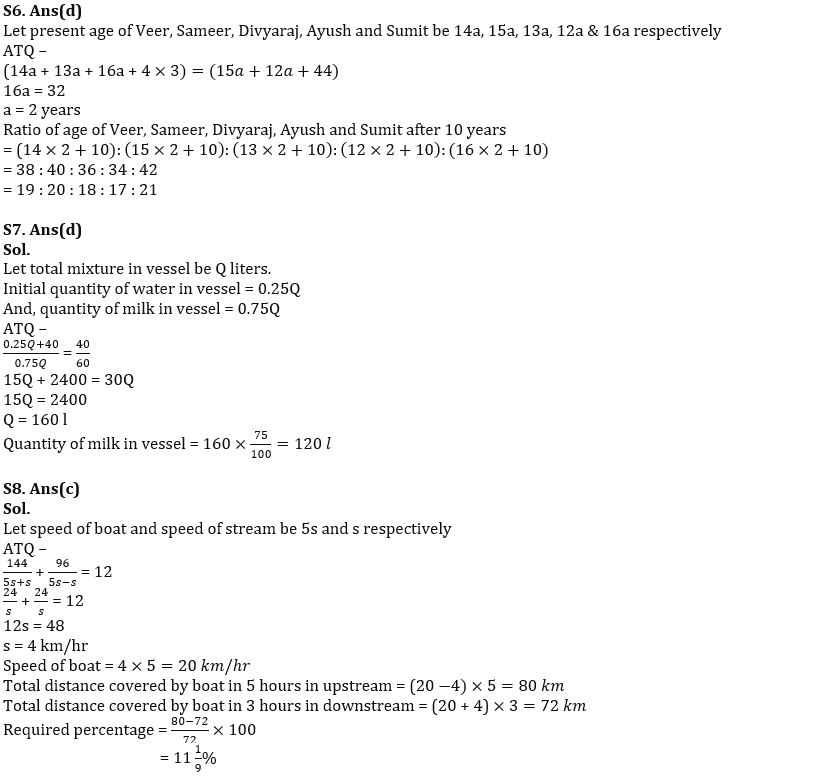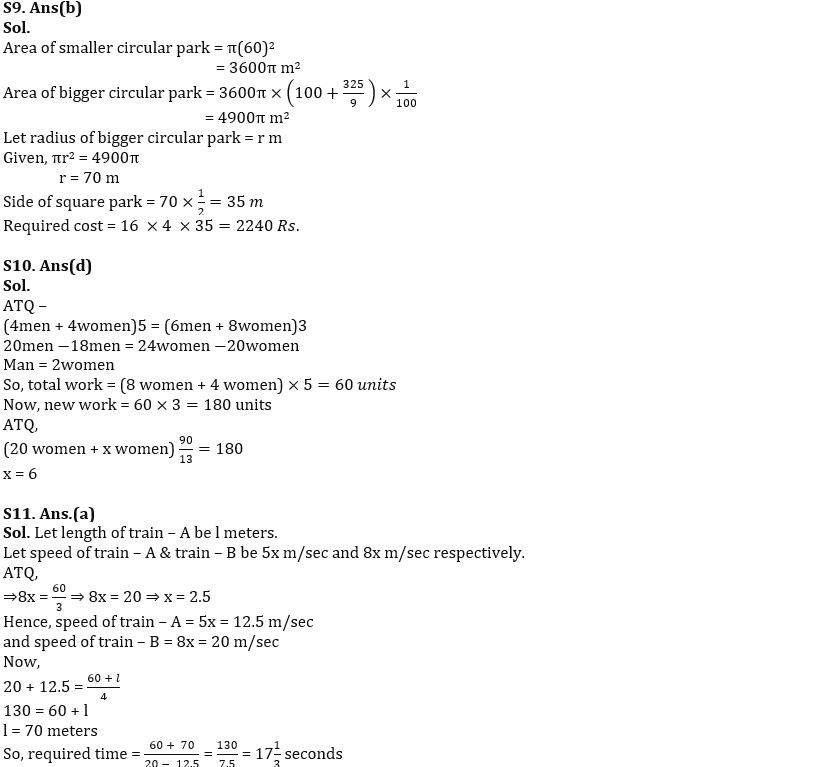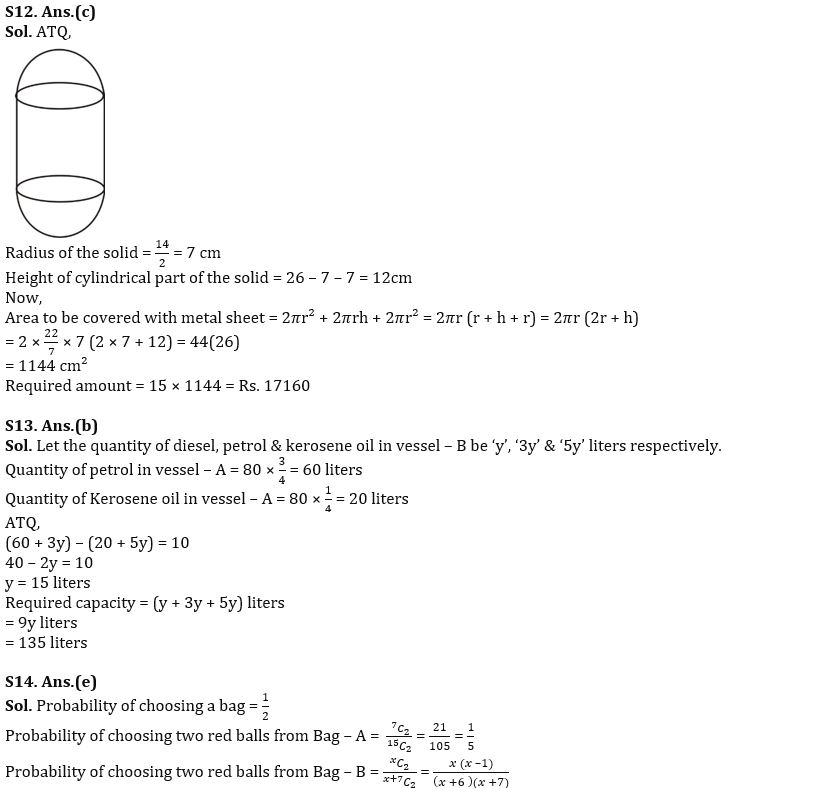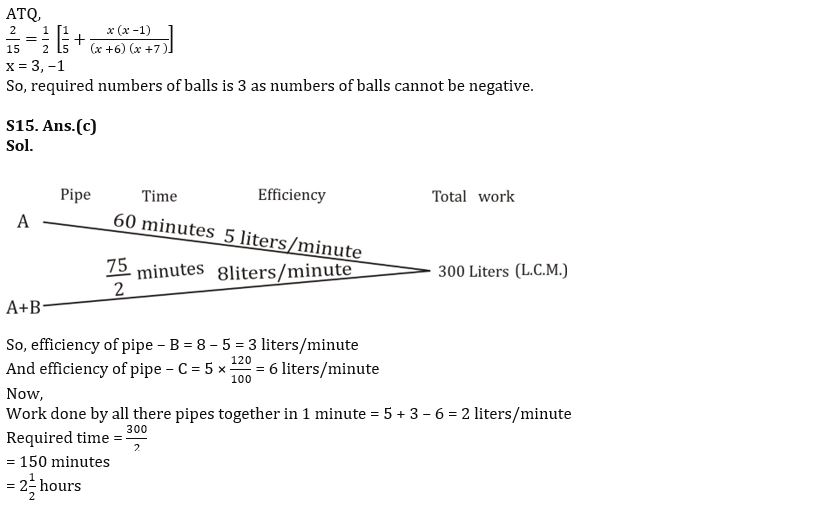#### Congratulations!Union Budget 2023-24: Free PDF